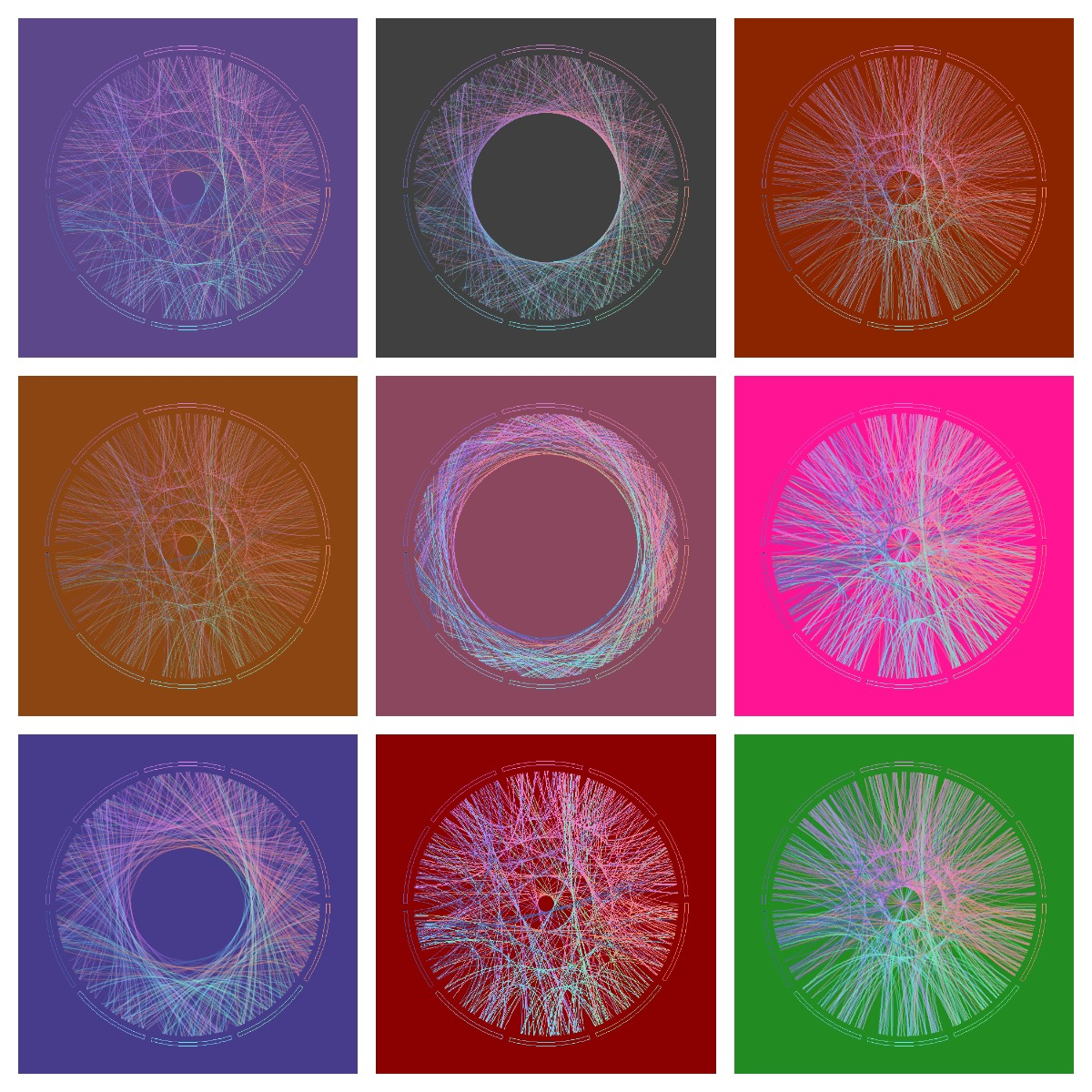# Discovering Shiny

It is not an experiment if you know it is going to work (Jeff Bezos)

From time to time, I discover some of my experiments translated into Shiny Apps, like this one. Some days ago, I discovered one of these translations and I contacted the author, who was a guy from Vietnam called Vu Anh. I asked him to do a Shiny App from this experiment. Vu was enthusiastic with the idea. We defined some parameters to play with shape, number, width and alpha of lines as well as background color and I received a perfect release of the application in just a few hours. With just a handful of parameters, possible outputs are almost infinite. Following you can find some of them:I think the code is a nice example to take the first steps in Shiny. If you are not used to Markdown files, you can follow this instructions to run the code.

Vu is a talented guy, who loves maths and programming. He represents the future of our nice profession and I predict a successful future for him. Do not miss his brand new blog. I am sure you will find amazing things there.

This is the code of the app:

```---
title: "Maths, Music and Merkbar"
author: "Brother Rain"
date: "18/03/2015"
output: html_document
runtime: shiny
---

```{r}
library(circlize)
library(scales)
factors = as.factor(0:9)
lines = 2000 #Number of lines to plot in the graph
alpha = 0.4  #Alpha for color lines
colors0=c(
rgb(239,143,121, max=255),
rgb(126,240,188, max=255),
rgb(111,228,235, max=255),
rgb(127,209,249, max=255),
rgb( 74,106,181, max=255),
rgb(114,100,188, max=255),
rgb(181,116,234, max=255),
rgb(226,135,228, max=255),
rgb(239,136,192, max=255),
rgb(233,134,152, max=255)
)
# You can find the txt file here:
```

## Visualization

```{r, echo=FALSE}
fluidPage(
fluidRow(
column(width = 4,
sidebarPanel(
sliderInput("lines", "Number of lines:", min=100, max=100000, step=100, value=500),
sliderInput("alpha", "Alpha:", min=0.01, max=1, step=0.01, value=0.4),
sliderInput("lwd", "Line width", min=0, max=1, step=0.05, value=0.2),
selectInput("background", "Background:",
c("Purple" = "mediumpurple4", "Gray" = "gray25", "Orange"="orangered4",
"Violet"="palevioletred4", "Green"="forestgreen", "Pink"="deeppink"), selected="Purple"),
sliderInput("h0", "h0:", min=0, max=0.4,
step=0.0005, value=0.1375),
sliderInput("h1", "h1:", min=0, max=0.4,
step=0.0005, value=0.1125),
width=12
)
),
column(width = 8,
renderPlot({
# get data
phi=gsub("\\.","", substr(phi,1,input\$lines))
phi=gsub("\\.","", phi)
position=1/(nchar(phi)-1)

# create circos
circos.clear()
par(mar = c(1, 1, 1, 1), lwd = 0.1,
cex = 0.7, bg=alpha(input\$background, 1))
circos.par(
"track.height" = 0.025,
"gap.degree" = 3
)
circos.initialize(factors = factors, xlim = c(0, 1))
circos.trackPlotRegion(factors = factors, ylim = c(0, 1))
## create first region
for (i in 0:9) {
circos.updatePlotRegion(
sector.index = as.character(i),
bg.col = alpha(input\$background, 1),
bg.border=alpha(colors0[i+1], 1)
)
}
for (i in 1:(nchar(phi)-1)) {
m=min(as.numeric(substr(phi, i, i)), as.numeric(substr(phi, i+1, i+1)))
M=max(as.numeric(substr(phi, i, i)), as.numeric(substr(phi, i+1, i+1)))
d=min((M-m),((m+10)-M))
col=t(col2rgb(colors0[(as.numeric(substr(phi, i, i))+1)]))
for(index in 1:3){
col[index] = max(min(255, col[index]), 0)
}
if (d>0) {
substr(phi, i, i), position*(i-1),
substr(phi, i+1, i+1), position*i,
h = input\$h0 * d + input\$h1,
lwd=input\$lwd,
col=alpha(rgb(col, max=255), input\$alpha), rou = 0.92
)
}
}
}, width=600, height=600, res=192)
)
)
)

```
```